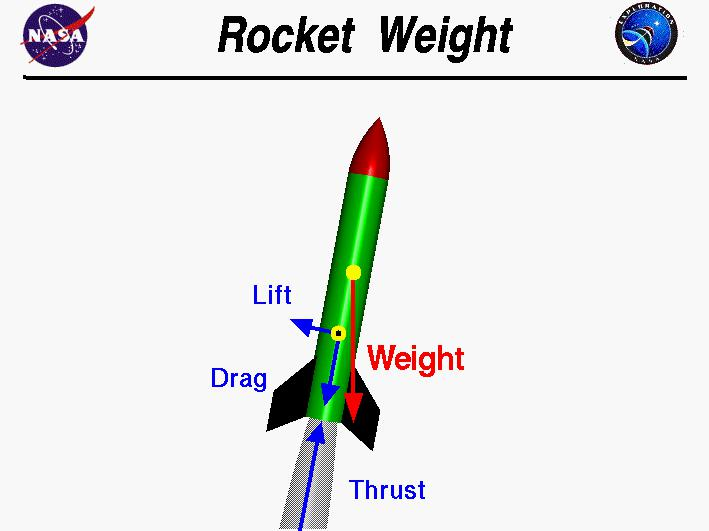# Calculating rocket acceleration — Science Learning Hub

how fast is a rocket ship
habboin 29/12/2021 Rocket 2098
How does the acceleration of a model rocket compare to the Space Shuttle? By using the resultant force and mass, acceleration can be calculated.Forces acting The two forces acting on rockets at the mo...

How does the acceleration of a model rocket compare to the Space Shuttle? By using the resultant force and mass, acceleration can be calculated.

## Forces acting

The two forces acting on rockets at the moment of launch are the thrust upwards and the weight downwards. Weight is the force due to gravity and is calculated (at the Earth’s surface) by multiplying the mass (kilograms) by 9.8.

The resultant force on each rocket is calculated using the equation resultant force = thrust – weight.

## Calculating the acceleration of a model rocket

Acceleration is a measure of how much the speed increases each second. It can be calculated using the equationacceleration = resultant force (newtons, N) divided by mass (kilograms, kg).

One model rocket has a mass of 50 grams and a rocket engine that produces a thrust of 5 N for 1 second.

To find the weight, 50 g needs to be changed into kilograms by dividing by 1000. This gives a mass of 0.050 kg. Weight is mass (in kg) x 9.8, which gives 0.050 x 9.8 = 0.49 N.

The resultant force is the thrust – weight = 5 – 0.49 = 4.51 N (unrounded).

Acceleration = resultant force divided by mass = 4.51 ÷ 0.050 = 90 metres per second squared (90 m/s2). This means that, every second, the speed of the rocket increases by 90 m/s.

This is nine times the normal acceleration due to gravity.The same method can be used for a full-sized rocket such as the Space Shuttle.

## Calculating the acceleration of the Space Shuttle

The Space Shuttle mission that launched on 24 February 2011 was called STS-133. It had a lift-off mass of 2 million kilograms (2000 tonnes). This means the total weight at lift-off is 2 million x 9.8, which is approximately 20 million newtons (20 mega newtons).

The thrust of each of the big white solid rocket boosters on the side was 12.5 million newtons, and the three main engines at the tail end of the bit that looks like a plane (the Space Shuttle orbiter) had a combined thrust of 5.5 million newtons. This gives a massive total thrust of 30.5 million newtons!

Resultant force = thrust – weight = 30.5 million – 20 million = 10.5 million newtons.

Acceleration = resultant force ÷ mass = 10.5 million ÷ 2 million = 5.25 m/s2.

## Comparing accelerations

The acceleration of the model rocket at 90 m/s2 is far greater than the Space Shuttle at 5.25 m/s2, but there is a significant difference in the motion after launch.

Firstly, the model rocket only had enough propellant for 1 second of thrust. After 1 second, it reaches a maximum speed of 90 m/s (ignoring drag and mass change), but after that 1 second of thrust, the model rocket starts to lose speed due to the weight and drag forces both acting in the opposite direction to its motion.

For the Space Shuttle, the solid rocket boosters (SRBs) have enough propellant to provide thrust for 124 s. This means that the space shuttle accelerates for more than 2 minutes before the solid rocket boosters separate. (As the shuttle uses its propellant, it also becomes much lighter, which increases acceleration.)

After 124 seconds, the shuttle has already reached an altitude of 45 km and is travelling at 1,380 m/s (4,973 km/h). At this time (once all of the fuel is consumed), the SRBs separate away from the external tank (the big orange part). Fuel continues to be pumped to the three main engines for another 6 minutes. Acceleration continues until the Space Shuttle orbiter eventually reaches a speed of 28,000 km/h and an altitude over 300 km. This allows it to orbit around the Earth.

## Related content

Explore further some of the ideas that are fundamental to understanding rockets:

## Activity ideas

Explore using the Rocket launch challenge interactive in the Rocket launch challenge activity.

Follow this activity with Investigating rocket motion. Students investigate the motion of a rocket using a spreadsheet with graphs of motion included. They adjust variables and investigate how height and speed are affected.